# virtualPollen

### Generation of virtual pollen curves

#### 2022-02-11

Summary

This document describes in detail the methods used to generate a virtual environmental driver with a given temporal autocorrelation, to be used as an input for a population model simulating synthetic pollen curves generated by virtual taxa with different life-traits (life-span and fecundity) and environmental niche features (niche position and breadth). We also describe how we generated a virtual sediment accumulation rate to aggregate the results of the population model to mimic taphonomic processes producing real pollen curves, and how we resampled virtual pollen data at different depth intervals. Finally, we present the code used to generate the 16 virtual taxa used for the analyses described in the paper.

IMPORTANT: An Rmarkdown version of this document can be found at: https://github.com/BlasBenito/EcologicalMemory.

#Generating a virtual environmental driver#

###Rationale###

To simulate virtual pollen curves with the population model described in section 2 a virtual driver representing changes in environmental conditions is required. This section explains how to generate such a driver as a time series with a given temporal autocorrelation simulating the temporal structure generally shown by observed environmental drivers.

###Generating a random walk###

The following steps are required to generate a random walk in R:

1. Generate a vector time with time values.

2. Re-sample with replacement the set of numbers (-1, 0, 1) as many times as values are in the time vector, to produce the vector moves (as in moves of a random walk).

3. Compute the cumulative sum of moves, which generates the random walk.

#sets a state for the generator of pseudo-random numbers
set.seed(1)
#defines the variable "time"
time <- 1:10000
#samples (-1, 0, 1) with replacement
moves <- sample(x=c(-1, 0, 1), size=length(time), replace=TRUE)
#computes the cumulative sum of moves
random.walk <- cumsum(moves)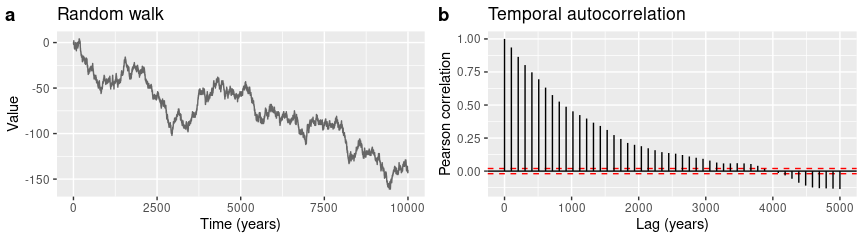Random walk (a) and its temporal autocorrelation (b).

Every time this code is executed with a different number in set.seed(), it generates a different random walk with a different temporal autocorrelation, but still, this simple method is not useful to generate a time series with a given temporal autocorrelation.

###Applying a convolution filter to generate a given temporal autocorrelation###

Applying a convolution filter to a time series allows to generate dependence among sets of consecutive cases. Below we show an example of how it works on a random sequence a composed by five numbers in the range [0, 1]. The operations to compute the filtered sequence are shown in Table 1.

#setting a fixed seed for the generator of pseudo-random numbers
set.seed(1)

#generating 5 random numbers in the range [0, 1]
a <- runif(5)

#applying a convolution filter of length 2 and value 1
b <- filter(a, filter=c(1,1), method="convolution", circular=TRUE)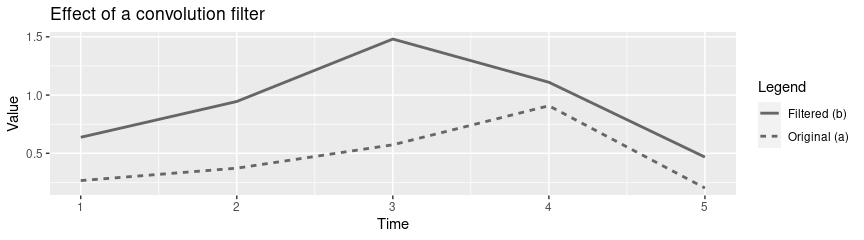Original sequence (dashed line) and filtered sequence with filter (solid line).

Original sequence (a), filtered sequence (b), and filtering operations. Numbers beside letters a and b represent row numbers, while f1 and f2 represent the values of the convolution filter (both equal to 1 in this case).
row a b operation
1 0.27 0.64 b1 = a1 x f2 + a2 x f1
2 0.37 0.94 b2 = a2 x f2 + a3 x f1
3 0.57 1.48 b3 = a3 x f2 + a4 x f1
4 0.91 1.11 b4 = a4 x f2 + a5 x f1
5 0.20 0.47 b5 = a5 x f2 + a6 x f1

The operation column in Table 1 shows how the convolution filter generates a dependence between values located within the length of the filter. This positional dependence creates a temporal autocorrelation pattern with a significant length equal to the length of the filter. The following piece of code demonstrates this by generating two versions of the same moves vector used before, one with a length of the significant temporal autocorrelation equal to 10 (defined in the same units of the time vector), and another with a length of 100. Results are shown in Figure 3.

moves.10 <- filter(moves, filter=rep(1, 10), circular=TRUE)
moves.100 <- filter(moves, filter=rep(1, 100), circular=TRUE)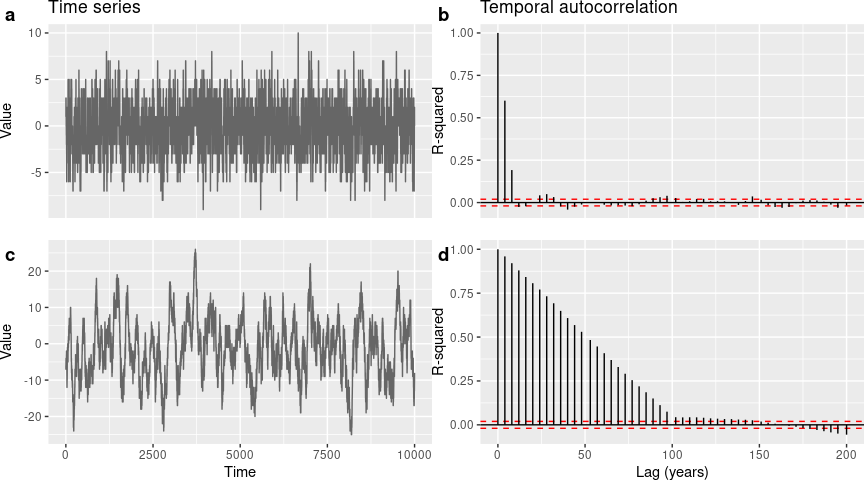Sequences filtered with different filter lengths: a) Sequence with autocorrelation length equal to 10; b) Temporal autocorrelation of a); c) Sequence with autocorrelation length equal to 100: d) Temporal autocorrelation of c).

A major limitation is that this method does not work well when the required length of the temporal autocorrelation is a large fraction of the overall length of the time series. The code below and Figure 4 show an example with a filter of length 5000 (note that the time variable has 10000 elements).

moves.5000 <- filter(moves, filter=rep(1, 5000), circular=TRUE)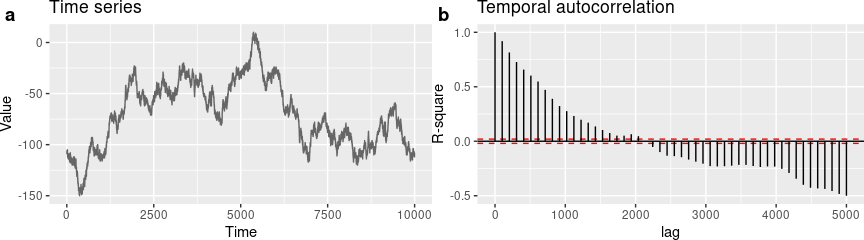Sequence moves.5000 (a) and its temporal autocorrelation (b). In this case there is a large deviation between the required temporal autocorrelation (5000) and the outcome (2000).

All the ideas presented above are implemented in the functions named simulateDriver() and simulateDriverS, with these main arguments:

• random.seed: an integer for set.seed(), to use the same random seed in the generation of random numbers and make sure that results are reproducible.
• age: a vector (i.e. 1:1000), representing the length of the output.
• autocorrelation.length: length of the desired temporal autocorrelation structure, in the same units as age.
• output.min: minimum value of the driver.
• output.max: maximum value of the driver.

Figure 5 shows a driver generated with simulateDriverS with annual resolution in the range [0, 100], over a period of 10000 years, with a temporal autocorrelation length of 600 years.

driver <- simulateDriverS(
random.seeds=c(60, 120),
time=1:10000,
autocorrelation.lengths = 600,
output.min=c(0, 0),
output.max=c(100, 100),
driver.names=c("A", "B"),
filename=NULL)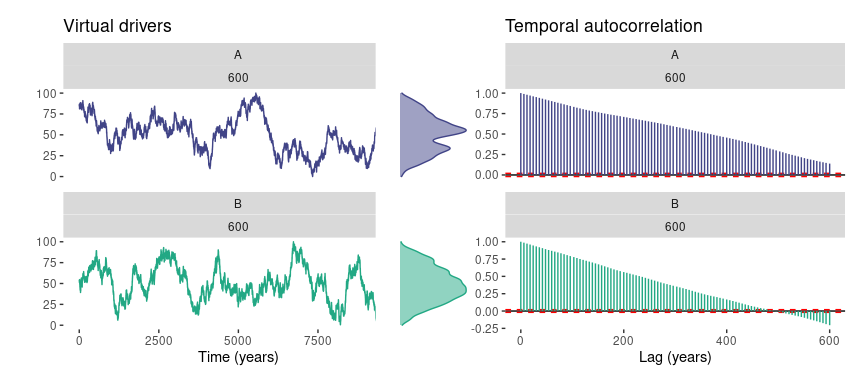Virtual driver and its temporal autocorrelation. Note that virtual driver B will not be used hereafter to simplify the explanation on the model dynamics.

#Simulating pollen curves from virtual taxa#

###Rationale###

The ability of plant populations to respond more or less quickly to environmental change is mediated by a set of species’ traits, such as niche optimum and breadth, growth rate, sexual maturity age, effective fecundity, and life span. Even though we have values for these traits for many plant species, pollen types are often clusters of species of the same genus or family rather than single species, making the assignment of trait values uncertain. For the purpose of this paper it is more practical to work with virtual pollen curves generated by virtual taxa with known relationships with the environment and traits. These virtual taxa are the result of a population model with different parametrizations.

###Assumptions###

The model follows these assumptions:

• The spatial structure of the population is not important to explain its pollen productivity. This is an operative assumption, to speed-up model executions. The lack of spatial structure is partially compensated by the model parameter charge capacity, which simulates a limited space for population expansion.
• The environmental niche of the species follows a Gaussian distribution, characterized by a mean (niche optimum, also niche position) and a standard deviation (niche breadth or tolerance).
• Different drivers can have a different influence on the species dynamics, and that influence can be defined by the user by tuning the weights of each driver.
• Environmental suitability, expressed in the range [0, 1], is the result of an additive function of the species niches (normal function defined by the species’ mean and standard deviation for each driver), the drivers’ values, and the relative influence of each driver (driver weights).
• Pollen productivity is a function of the individual’s biomass and environmental suitability, so under a hypothetical constant individual’s biomass, its pollen production depends linearly on environmental suitability values.
• Effective fecundity is limited by environmental suitability. Low environmental suitability values limit recruitment, acting as an environmental filter. Therefore, even though the fecundity of the individuals is fixed by the fecundity parameter, the overall population fecundity is limited by environmental suitability.

###Parameters###

We have designed a simple individual-based population model which input parameters are:

• Drivers: Values of up to two drivers (provided as numeric vectors) for a given time span.
• Niche mean: The average (in drivers units) of the normal functions describing the species niche for each driver. This parameter defines the niche optimum of the species.
• Niche breadth: Standard deviations (in driver units) of the normal functions describing the species niche for each driver. Smaller values simulate more specialized species, while larger values simulate generalist species.
• Driver weight: Importance of each driver (note that in the paper we only used one driver) in defining climatic suitability for the given species. Balanced weights mean each driver is contributing equally to climatic suitability. The sum of both drivers must be 1.
• Maximum age: age (in years) of senescence of the individuals. Individuals die when reaching this age.
• Reproductive age: age (in years) at which individuals start to produce pollen and seeds.
• Fecundity: actually, effective fecundity, which is the maximum number of viable seeds produced by mature individuals per year under under fully suitable climatic conditions.
• Growth rate: amount of the maximum biomass gained per year by each individual. Used as input in the logistic equation of the growth model.
• Maximum biomass: maximum biomass (in relative units) individuals can reach. Used as input in the logistic equation of the growth model to set a maximum growth.
• Carrying capacity: maximum sum of the biomass of all individuals allowed in the model. Ideally, to keep model performance, it should be equal to maximum biomass multiplied by 100 or 1000. The model removes individuals at random when this number is reached is reached.

In order to explain the model dynamics in the most simplified way, the parameters in Table 1 are considered.

Parameters of a virtual species. Note that driver.B is ommited in order to simplify the explanation of the model.
Parameter Species 1
1 1 50
2 2 20
3 3 2
4 4 0.2
5 5 0
6 6 100
7 7 10000
8 8 1
10 10 50
11 11 10

###Ecological niche and environmental suitability###

The model assumes that normal distributions can be used as simple mathematical representations of ecological niches. Considering a virtual species and an environmental predictor, the abundance of the species along the range of the predictor values can be defined by a normal distribution with a mean $$μ$$ (niche optimum or niche position), and standard deviation $$σ$$ (niche breadth or tolerance).

The equation to compute the density of a normal distribution has the form:

Equation 1: $f(x) = 1/(√(2 π) σ) e^{-((x - μ)^2/(2 σ^2))}$

Where:

• $$x$$ is the value of the predictor.
• $$μ$$ is the mean of the normal distribution.
• $$σ$$ is the standard deviation.

The following code shows a simple example on how dnorm() uses Equation 1 to compute the density of a normal function over a data range from a mean and a standard deviation:

niche.A <- dnorm(x=0:100, mean=50, sd=10)

We use the code above and a computation on the density of the driver to plot the ecological niche of the virtual taxa against the availability of driver values (Figure 6).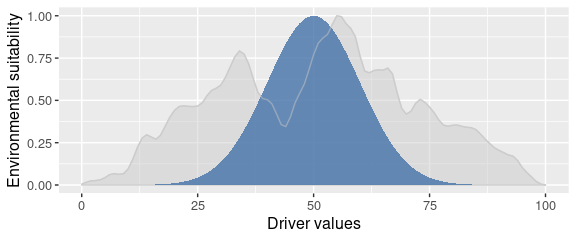Ecological niche of the virtual species (blue) against the density (relative availability of values over time) of the driver (gray). Both densities have been scaled in the range [0, 1].

Environmental suitability for the given species over the study period is computed as follows:

1. dnorm() is computed on the mean and standard deviation defined for the species niche for a given driver.
2. The output of dnorm() is scaled to the range [0, 1].
3. The scaled values of the output are multiplied by the driver weights (which equals 1 if only one driver is used).
4. If there are two drivers, suitability values of each individual driver are summed together.

A burn-in period with a length of ten generations of the virtual taxa is added to the environmental suitability computed from the species niche and the driver/drivers. The added segment starts at maximum environmental suitability, stays there for five generations, and decreases linearly for another five generations until meeting the first suitability value of the actual simulation time. The whole burn-in segment has a small amount of white noise added (Figure 7). The burn-in period lets the population model to warm-up and go beyond starting conditions before simulation time starts.

## Warning: Use of plot.df4\$Time is discouraged. Use Time instead.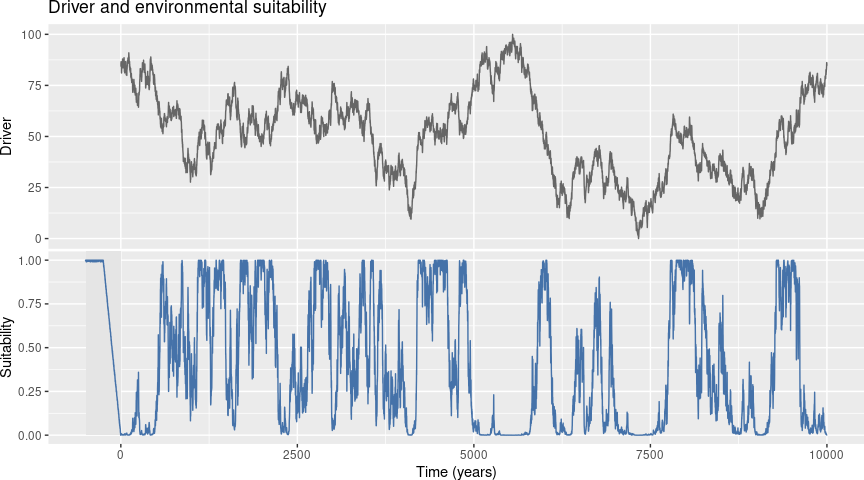Driver and environmental suitability of the virtual taxa. Burn-in period is highlighted by a gray box in the Environmental suitability panel.

###Biomass growth###

Individuals age one year on each simulation step, and their biomass at any given age is defined by the following equation of logistic growth (Equation 2). Figure 8 shows different growth curves for different growth rates for a virtual taxon with a maximum age of 400 years.

Equation 2: $f(x) = \frac{B}{1 + B} \times e^{(- \alpha \times t)}$

Where:

• $$B$$ is the maximum biomass an individual can reach.
• $$\alpha$$ is the growth rate.
• $$t$$ is the age of the individual at a given time.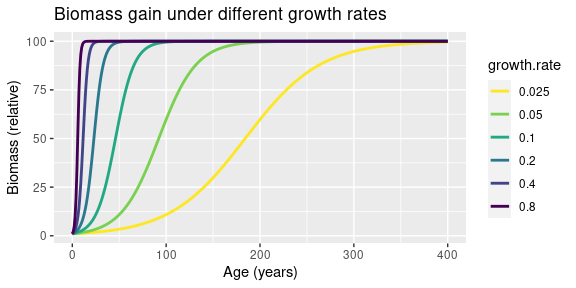Biomass vs. age curves resulting from different growth rates for a 400 years life-span.

###Population dynamics###

The model starts with a population of 100 individuals with random ages, in the range [1, maximum age], taken from a uniform distribution (all ages are equiprobable). For each environmental suitability value, including the burn-in period, the model performs the following operations:

1. Aging: adds one year to the age of the individuals.
2. Mortality due to senescence: individuals reaching the maximum age are removed from the simulation.
• Local extinction and immigration If the number of individuals drops to zero, the population is replaced by a “seed bank” of 100 individuals with age zero, and the simulation jumps to step 7.. This is intended to simulate the arrival of seeds from nearby regions, and will only lead to population growth if environmental suitability is higher than zero.
3. Plant growth: Applies a plant growth equation to compute the biomass of every individual (see Figure 8).
4. Carrying capacity: If maximum population biomass is reached, individuals are iteratively selected for removal according to a mortality risk curve computed by Equation 3 (see Figure 9). This curve gives removal preference to younger individuals, matching observed patterns in natural populations.
5. Pollen productivity: In each time step the model computes the pollen productivity (in relative values) of the population using Equation 4.
6. Reproduction: Generates as many seeds as reproductive individuals are available multiplied by the maximum fecundity and the environmental suitability of the given time.

The model returns a table with climatic suitability, pollen production, and population size (reproductive individuals only) per simulation year. Figure 10 shows the results of the population model when applied to the example virtual species.

Equation 3: $P_{m} = 1 - sqrt(a/A)$

Where:

• $$P_{m}$$ is the probability of mortality.
• $$a$$ is the age of the given individual.
• $$A$$ is the maximum age reached by the virtual taxa.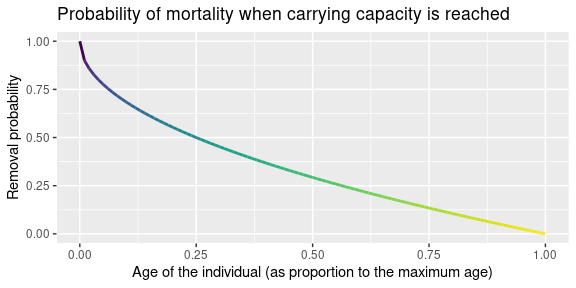Risk curve defining the probability of removal of a given individual as a function of its fractional age when maximum carrying capacity is reached.

Equation 4: $P_{t} = \sum x_{it} \times max(S_{t}, B)$

Where:

• $$t$$ is time (a given simulation time step).
• $$P$$ is the pollen productivity of the population at a given time.
• $$x_{i}$$ represents the biomass of every adult individual.
• $$S$$ is the environmental suitability at the given time.
• $$B$$ is the contribution of biomass to pollen productivity regardless of environmental suitability (pollen.control parameter in the simulation, 0 by default). If $$B$$ equals 1, $$P$$ is equal to the total biomass sum of the adult population, regardless of the environmental suitability. If $$B$$ equals 0, pollen productivity depends entirely on environmental suitability values.

The code below shows the core of the simulatePopulation function. It is slightly simplified to improve readability, and only pollen counts are written as output. Note that age of individuals is represented as a proportion of the maximum age to facilitate operations throughout the code.

#parameters (1st line in dataframe "parameters")
maximum.age <- parameters[1, "maximum.age"]
reproductive.age <- parameters[1, "reproductive.age"]
growth.rate <- parameters[1, "growth.rate"]
carrying.capacity <- parameters[1, "carrying.capacity"]
fecundity <- parameters[1, "fecundity"]

#reproductive age to proportion
reproductive.age <- reproductive.age / maximum.age

#years scaled taking maximum.age as reference
scaled.year <- 1/maximum.age

#vector to store pollen counts
pollen.count <- vector()

#starting population
population <- sample(seq(0, 1, by=scaled.year),
100,
replace=TRUE)

#iterating through suitability (once per year)
#------------------------------------
for(suitability.i in suitability){

#AGING -----------------------------------------------
population <- population + scaled.year

#SENESCENCE ------------------------------------------
#1 is the maximum age of ages expressed as proportions
population <- population[population < 1]

#LOCAL EXTINCTION AND RECOLONIZATION -----------------
if (length(population) == 0){

#local extinction, replaces population with a seedbank
population <- rep(0, floor(100 * suitability.i))

#adds 0 to pollen.count
pollen.count <- c(pollen.count, 0)

#jumps to next iteration
next
}

#PLANT GROWTH ---------------------------------------
#biomass of every individual
biomass <- maximum.biomass /
(1 +  maximum.biomass *
exp(- (growth.rate * suitability.i) *
(population * maximum.age)
)
)

#SELF-THINNING --------------------------------------
#carrying capacity reached
while(sum(biomass) > carrying.capacity){

#removes a random individual based on risk curve
individual.to.remove <- sample(
x = length(population),
size = 1,
replace = TRUE,
prob = 1 - sqrt(population) #risk curve
)

#removing individuals from population and biomass
population <- population[-individual.to.remove]
biomass <- biomass[-individual.to.remove]

}#end of while

#REPRODUCTION --------------------------------------
adults <- population > reproductive.age

#seeds (vector of 0s)
#fractional biomass of adults * fecundity * suitability
seeds <- rep(0, floor(sum((biomass[adults]/maximum.biomass) *
fecundity) * suitability.i))

#adding seeds to the population
population <- c(population, seeds)

#POLLEN OUTPUT -------------------------------------
#biomass of adults multiplied by suitability
pollen.count <- c(pollen.count,

} #end of loop through suitability values

The code below executes the simulation, and plots the outcome using the function plotSimulation.

#simulate population based on parameters
simulation <- simulatePopulation(parameters=parameters[1, ],
drivers=driver)

#plotting simulation output
plotSimulation(simulation.output=simulation,
burnin=FALSE,
panels=c("Driver A",
"Suitability",
"Population",
"Pollen"),
plot.title="",
text.size=12,
line.size=0.4)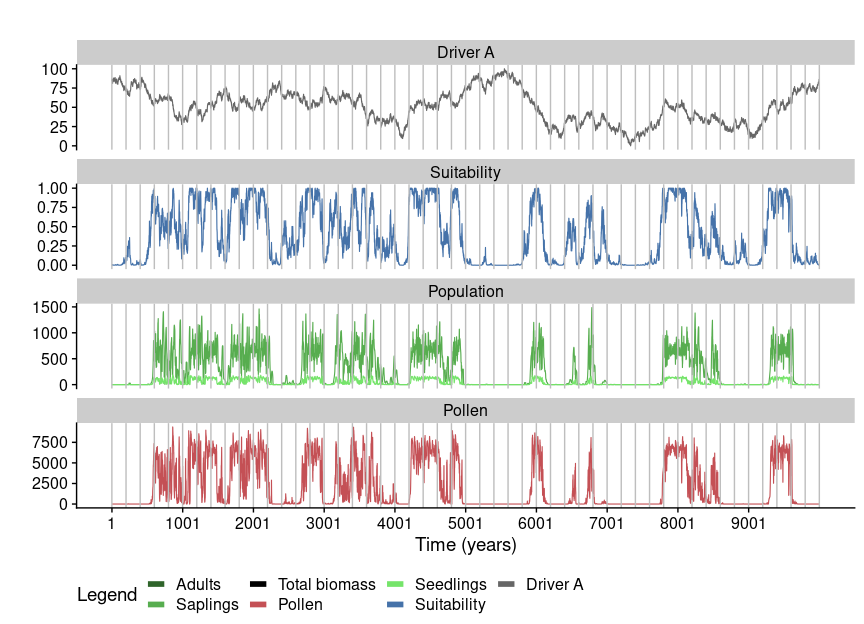Simulation outcome. Green shades represent different age groups (seedlings, saplings, and adults).

The simulation outcomes can vary with the traits of the virtual species. Table 2 shows the parameters of two new taxa named Species 2 and Species 3. These species have a higher niche breadth than Species 1, and Species 3 has a pollen productivity depending more on biomass than suitability (parameter pollen.control higher than zero). The comparison of both simulations (Figure 11) along with Species 1 shows that different traits generate different pollen curves in our simulation.

Parameters of the three virtual species.
Parameter Species 1 Species 2 Species 3
maximum.age 50 50 50
reproductive.age 20 20 20
fecundity 2 4 6
growth.rate 0.2 0.3 0.4
pollen.control 0.0 0.0 0.5
maximum.biomass 100 100 100
carrying.capacity 10000 10000 10000
driver.A.weight 1 1 1
niche.A.mean 50 50 50
niche.A.sd 10 15 20
#simulating species 2 and 3 of the dataframe parameters
simulation.2 <- simulatePopulation(parameters=parameters,
species=c(2,3),
drivers=driver)

#adding the results to the previous simulation
simulation <- c(simulation, simulation.2)
rm(simulation.2)

#plotting the comparison for the time interval between 4000 and 5000.-
compareSimulations(simulation.output=simulation,
species = "all",
columns = c("Suitability", "Pollen"),
time.zoom = c(5600, 6400))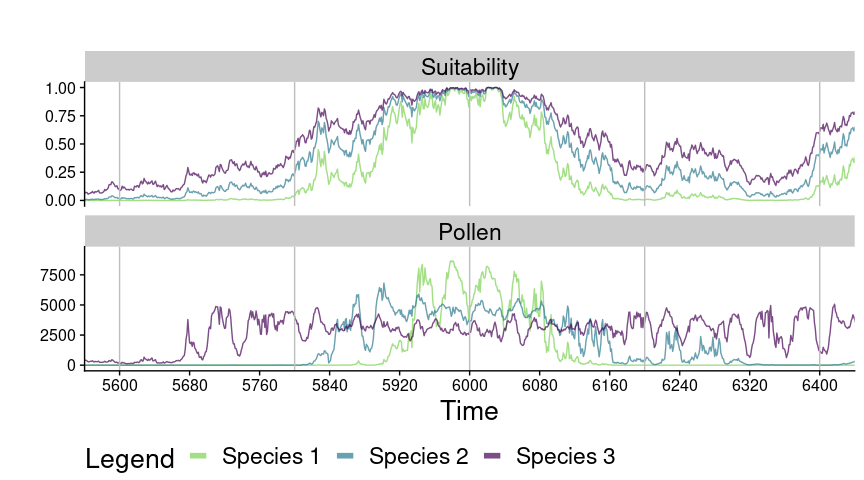Comparison of the pollen abundance and environmental suitability (same in all cases) for the three virtual species shown in Table 2 within the period 5600-6400. Species 2 has a higher fecundity than Species 1 (1 vs 10)

###Testing the model limits###

We searched for the minimum values of the parameters required to keep a simulated population viable under fully suitable conditions. The taxa Test 1 and Test 2 shown below

Parameters of virtual taxa used to test model limits.
Parameter Test 1 Test 2
maximum.age 4 3
reproductive.age 1 1
fecundity 0.55 0.50
growth.rate 2 2
pollen.control 0 0
maximum.biomass 1 1
carrying.capacity 30 30
driver.A.weight 0.5 0.5
driver.B.weight 0.5 0.5
niche.A.mean 50 50
niche.A.sd 10 10
niche.B.mean 50 50
niche.B.sd 10 10
simulation.test.1 <- simulatePopulation(
parameters=parameters.test,
driver.A=jitter(rep(50, 500), amount=4)
)

The test driver used had an average of 50 (optimum values according the environmental niche of both species) for 500 years, with random noise added through the jitter function. The model results (column Pollen only) for both virtual taxa are shown below.

compareSimulations(simulation.output=simulation.test.1,
columns="Pollen",
time.zoom = c(0, 200))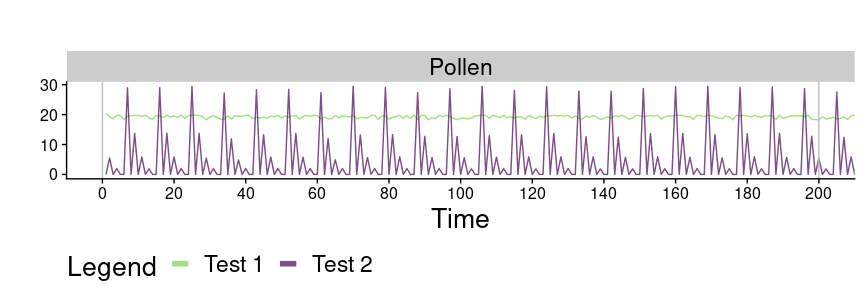Pollen output of virtual taxa Test 1 and Test 2 for a 200 years time-window.

The outputs of the test taxa show how a minimal change in the parameters can lead to fully unstable results when the considered taxa are short lived. Considering such a result, values for life-traits (maximum.age, reproductive.age, fecundity, growth.rate, and maximum.biomass) of taxon Test 1 should be taken as safe lower limits for these traits.

A similar situation can happen with long lived species when the age of sexual maturity is close to the maximum age. The table below shows three new test species with long life-spans and increasing maturity ages. The driver is again centered in 50, with added white noise, and 2000 years length.

Parameters of virtual taxa used to test model limits.
Parameter Test 3 Test 4 Test 5
maximum.age 1000 1000 1000
reproductive.age 100 500 900
fecundity 0.5 0.5 0.5
growth.rate 0.05 0.05 0.05
pollen.control 0 0 0
maximum.biomass 100 100 100
carrying.capacity 10000 10000 10000
driver.A.weight 0.5 0.5 0.5
driver.B.weight 0.5 0.5 0.5
niche.A.mean 50 50 50
niche.A.sd 10 10 10
niche.B.mean 50 50 50
niche.B.sd 10 10 10
simulation.test.2 <- simulatePopulation(
parameters=parameters.test,
species=c(3:5),
driver.A=jitter(rep(50, 2000), amount=4)
)
compareSimulations(simulation.output=simulation.test.2,
columns="Pollen",
time.zoom = c(0, 800))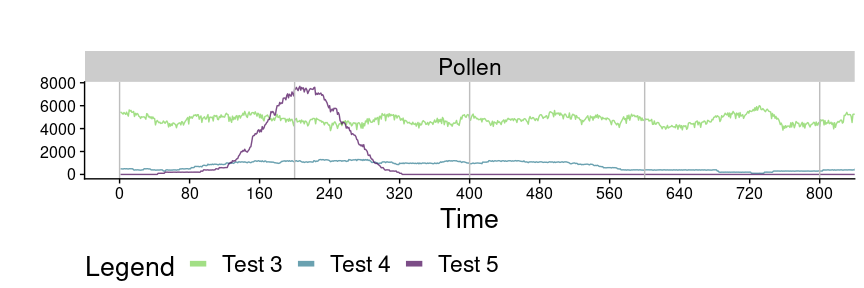Pollen output of virtual taxa Test 1 and Test 2 for a 200 years time-window.

The figure shows how Test 3 yields a stable pollen productivity across time, while Test 4 and Test 5 show, respectively, a very low productivity due to scarcity of adults, a total inability to sustain stable populations. Considering these results, it is important to keep a careful balance between the parameters maximum.age and reproductive.age to obtain viable virtual populations.

#Simulating a virtual accumulation rate#

Sediments containing pollen grains accumulate at varying rates, generally measured in years per centimeter (y/cm). Accumulation rates found in real datasets are broadly between 10 and 70 y/cm, with a paulatine increase towards the present time. To simulate such an effect and aggregate the annual data produced by the simulation in a realistic manner we have written a function named simulateAccumulationRate that takes a random seed, a time-span, and a range of possible accumulation rate values, and generates a virtual accumulation rate curve. It does so by generating a random walk first, smoothing it through the application of a GAM model, and scaling it between given minimum and maximum accumulation rates (see Figure 12).

accumulation.rate <- simulateAccumulationRate(seed=140,
time=1:10000,
output.min=1,
output.max=50)